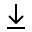MathBin Quadrilateral Member

A quadrilateral is a two-dimensional object that exists in two dimensions or higher. A quadrilateral is a polygon with four edges (sides) and four vertices (angles).

Quadrilaterals are simple (not self-intersecting) or complex (self-intersecting), also called crossed. Simple quadrilaterals are either convex or concave. The interior angles of a simple (and planar) quadrilateral ABCD add up to 360 degrees.

The area of a simple quadrilateral given its vertices P1, P2, P3, P4 is Area = [(x1y2 - y1x2) + (x2y3 - y2x3) + (x3y4 - y3x4) + (x4y1 - y4x1)] / 2

Bins in Two Dimensions